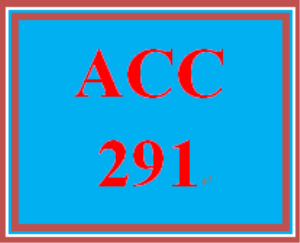# ACC 291 Week 3 Practice Quiz

PLDZ-4907 In Stock
\$ 7.00 USD
Description

ACC 291 Week 3 Practice Quiz

http://issuu.com/lucky269/docs/uoptutorial.docx

# ACC 291 Week 3 Practice Quiz

Practice Question 01

The time period for classifying a liability as current is one year or the operating cycle, whichever is

probable.

longer.

shorter.

possible.

Practice Question 05

Which one of the following is not a typical current liability?

Mortgages payable

Salaries payable

Current maturities of long-term debt

Interest payable

Practice Question 10

Buttner Company borrows \$88,500 on September 1, 2017, from Harrington State Bank by signing an \$88,500, 12%, one-year note. How much is accrued interest at December 31, 2017?

\$10,620

\$2,655

\$3,540

\$4,425

Practice Question 25

How is the market value of a bond issuance determined?

By adding the face value of the principal amount to the stated value of the interest payments.

By computing the present value of the principal.

By computing the present value of the interest payments.

By adding the present value of the principal amount to the present value of the interest payments.

Practice Question 30

If the contractual rate of interest is lower than the market rate of interest, bonds will sell at a premium.

True

False

Practice Question 35

What is the effect of amortizing a bond discount?

It decreases bond interest expense.

It increases the carrying value of the bonds.

It decreases the maturity value of the bonds.

There is no effect on the bond interest expense.

Practice Question 31

Cuso Inc. issues 10-year bonds with a maturity value of \$200,000. If the bonds are issued at a premium, what does this indicate?

The contractual interest rate exceeds the market interest rate.

The contractual interest rate and the market interest rate are the same.

The market interest rate exceeds the contractual interest rate.

No relationship exists between the market and contractual rates.

Practice Question 34

When a bond is sold at a premium, at what amount is it reported on the balance sheet?

Market value

Interest value

Carrying value

Practice Question 36

Tanner, Inc. issued a 10%, 5-year, \$100,000 bond when the market rate of interest was 12%. At what value will the bond sell?

Face value

A discount

Par

Practice Question 46

Which of the following is not a commonly used method of presenting current liabilities on the balance sheet?

Listing currently maturing long-term debt first.

Listing current debt in the order of oldest first and then chronologically.

In order of magnitude or size.

In order of their maturity.

Recent Reviews Write a Review
0 0 0 0 reviews